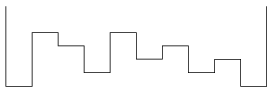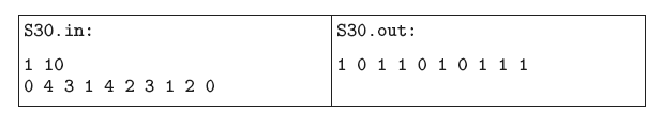Mathematical and Physical Journal
for High Schools
Issued by the MATFUND Foundation
 Already signed up? New to KöMaL?

#Problem S. 30. (November 2007)

S. 30. We would like to determine where puddles and pools are formed if the amount of precipitation is given. For simplicity, we consider only the cross-section of the given region, which is divided into horizontal line segments of fixed length with known relative altitudes. We can suppose that the distribution of precipitation is uniform.Water flows from higher areas to the neighbouring lower ones. If a segment has two lower neighbours, then the amount of water flowing down is equally divided between those neighbours. Water on the boundary segments can only flow inward.

Write your program to determine where water finally accumulates after the rain.

The input of your program is file s30.in. The first line of this file contains 2 integers separated by a space describing the amount of precipitation in millimeters and the number N of segments (). The second line contains N integers representing the height of each segment in millimeters relative to the lowest one.

The output of your program is s30.out, containing a single line with N digits separated by spaces. The ith digit is 1, if the ith segment contains water finally, otherwise 0.

The example corresponding to the above figure is the following:(10 pont)

Deadline expired on December 17, 2007.

Sorry, the solution is available only in Hungarian. Google translation

Megoldás

A feladat mintamegoldásaként egy Pascal programot közlünk:

A benne lévő kommentek alapján könnyen érthető a müködése.

### Statistics:

 4 students sent a solution. 10 points: Fábián András, Szebeni Szilveszter. 8 points: 2 students.

Problems in Information Technology of KöMaL, November 2007